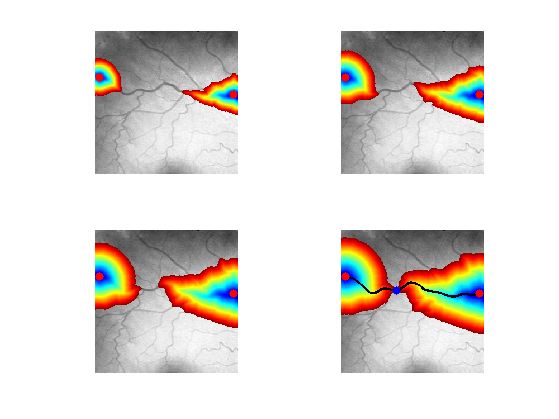$\newcommand{\NN}{\mathbb{N}} \newcommand{\CC}{\mathbb{C}} \newcommand{\GG}{\mathbb{G}} \newcommand{\LL}{\mathbb{L}} \newcommand{\PP}{\mathbb{P}} \newcommand{\QQ}{\mathbb{Q}} \newcommand{\RR}{\mathbb{R}} \newcommand{\VV}{\mathbb{V}} \newcommand{\ZZ}{\mathbb{Z}} \newcommand{\FF}{\mathbb{F}} \newcommand{\KK}{\mathbb{K}} \newcommand{\UU}{\mathbb{U}} \newcommand{\EE}{\mathbb{E}} \newcommand{\Aa}{\mathcal{A}} \newcommand{\Bb}{\mathcal{B}} \newcommand{\Cc}{\mathcal{C}} \newcommand{\Dd}{\mathcal{D}} \newcommand{\Ee}{\mathcal{E}} \newcommand{\Ff}{\mathcal{F}} \newcommand{\Gg}{\mathcal{G}} \newcommand{\Hh}{\mathcal{H}} \newcommand{\Ii}{\mathcal{I}} \newcommand{\Jj}{\mathcal{J}} \newcommand{\Kk}{\mathcal{K}} \newcommand{\Ll}{\mathcal{L}} \newcommand{\Mm}{\mathcal{M}} \newcommand{\Nn}{\mathcal{N}} \newcommand{\Oo}{\mathcal{O}} \newcommand{\Pp}{\mathcal{P}} \newcommand{\Qq}{\mathcal{Q}} \newcommand{\Rr}{\mathcal{R}} \newcommand{\Ss}{\mathcal{S}} \newcommand{\Tt}{\mathcal{T}} \newcommand{\Uu}{\mathcal{U}} \newcommand{\Vv}{\mathcal{V}} \newcommand{\Ww}{\mathcal{W}} \newcommand{\Xx}{\mathcal{X}} \newcommand{\Yy}{\mathcal{Y}} \newcommand{\Zz}{\mathcal{Z}} \newcommand{\al}{\alpha} \newcommand{\la}{\lambda} \newcommand{\ga}{\gamma} \newcommand{\Ga}{\Gamma} \newcommand{\La}{\Lambda} \newcommand{\Si}{\Sigma} \newcommand{\si}{\sigma} \newcommand{\be}{\beta} \newcommand{\de}{\delta} \newcommand{\De}{\Delta} \renewcommand{\phi}{\varphi} \renewcommand{\th}{\theta} \newcommand{\om}{\omega} \newcommand{\Om}{\Omega} \renewcommand{\epsilon}{\varepsilon} \newcommand{\Calpha}{\mathrm{C}^\al} \newcommand{\Cbeta}{\mathrm{C}^\be} \newcommand{\Cal}{\text{C}^\al} \newcommand{\Cdeux}{\text{C}^{2}} \newcommand{\Cun}{\text{C}^{1}} \newcommand{\Calt}{\text{C}^{#1}} \newcommand{\lun}{\ell^1} \newcommand{\ldeux}{\ell^2} \newcommand{\linf}{\ell^\infty} \newcommand{\ldeuxj}{{\ldeux_j}} \newcommand{\Lun}{\text{\upshape L}^1} \newcommand{\Ldeux}{\text{\upshape L}^2} \newcommand{\Lp}{\text{\upshape L}^p} \newcommand{\Lq}{\text{\upshape L}^q} \newcommand{\Linf}{\text{\upshape L}^\infty} \newcommand{\lzero}{\ell^0} \newcommand{\lp}{\ell^p} \renewcommand{\d}{\ins{d}} \newcommand{\Grad}{\text{Grad}} \newcommand{\grad}{\text{grad}} \renewcommand{\div}{\text{div}} \newcommand{\diag}{\text{diag}} \newcommand{\pd}{ \frac{ \partial #1}{\partial #2} } \newcommand{\pdd}{ \frac{ \partial^2 #1}{\partial #2^2} } \newcommand{\dotp}{\langle #1,\,#2\rangle} \newcommand{\norm}{|\!| #1 |\!|} \newcommand{\normi}{\norm{#1}_{\infty}} \newcommand{\normu}{\norm{#1}_{1}} \newcommand{\normz}{\norm{#1}_{0}} \newcommand{\abs}{\vert #1 \vert} \newcommand{\argmin}{\text{argmin}} \newcommand{\argmax}{\text{argmax}} \newcommand{\uargmin}{\underset{#1}{\argmin}\;} \newcommand{\uargmax}{\underset{#1}{\argmax}\;} \newcommand{\umin}{\underset{#1}{\min}\;} \newcommand{\umax}{\underset{#1}{\max}\;} \newcommand{\pa}{\left( #1 \right)} \newcommand{\choice}{ \left\{ \begin{array}{l} #1 \end{array} \right. } \newcommand{\enscond}{ \left\{ #1 \;:\; #2 \right\} } \newcommand{\qandq}{ \quad \text{and} \quad } \newcommand{\qqandqq}{ \qquad \text{and} \qquad } \newcommand{\qifq}{ \quad \text{if} \quad } \newcommand{\qqifqq}{ \qquad \text{if} \qquad } \newcommand{\qwhereq}{ \quad \text{where} \quad } \newcommand{\qqwhereqq}{ \qquad \text{where} \qquad } \newcommand{\qwithq}{ \quad \text{with} \quad } \newcommand{\qqwithqq}{ \qquad \text{with} \qquad } \newcommand{\qforq}{ \quad \text{for} \quad } \newcommand{\qqforqq}{ \qquad \text{for} \qquad } \newcommand{\qqsinceqq}{ \qquad \text{since} \qquad } \newcommand{\qsinceq}{ \quad \text{since} \quad } \newcommand{\qarrq}{\quad\Longrightarrow\quad} \newcommand{\qqarrqq}{\quad\Longrightarrow\quad} \newcommand{\qiffq}{\quad\Longleftrightarrow\quad} \newcommand{\qqiffqq}{\qquad\Longleftrightarrow\qquad} \newcommand{\qsubjq}{ \quad \text{subject to} \quad } \newcommand{\qqsubjqq}{ \qquad \text{subject to} \qquad }$

Fast Marching in 2D

# Fast Marching in 2D

This tour explores the use of Fast Marching methods in 2-D.

## Installing toolboxes and setting up the path.

You need to download the following files: signal toolbox, general toolbox and graph toolbox.

You need to unzip these toolboxes in your working directory, so that you have toolbox_signal, toolbox_general and toolbox_graph in your directory.

For Scilab user: you must replace the Matlab comment '%' by its Scilab counterpart '//'.

Recommandation: You should create a text file named for instance numericaltour.sce (in Scilab) or numericaltour.m (in Matlab) to write all the Scilab/Matlab command you want to execute. Then, simply run exec('numericaltour.sce'); (in Scilab) or numericaltour; (in Matlab) to run the commands.

Execute this line only if you are using Matlab.

getd = @(p)path(p,path); % scilab users must *not* execute this


Then you can add the toolboxes to the path.

getd('toolbox_signal/');
getd('toolbox_general/');
getd('toolbox_graph/');


## Shortest Path for Isotropic Metrics

Shortest paths are 2D curves that minimize a weighted length according to a given metric $$W(x)$$ for $$x \in [0,1]^2$$. The metric is usually computed from an input image $$f(x)$$.

The length of a curve $$t \in [0,1] \mapsto \gamma(t) \in [0,1]^2$$ is $L(\gamma) = \int_0^1 W(\gamma(t)) \norm{\gamma'(t)} \text{d} t.$

Note that $$L(\gamma)$$ is invariant under re-parameterization of the curve $$\gamma$$.

A geodesic curve $$\gamma$$ between two points $$x_0$$ and $$x_1$$ has minimum length among curves joining $$x_0$$ and $$x_1$$, $\umin{\ga(0)=x_0, \ga(1)=x_1} L(\ga).$ A shortest curve thus tends to pass in areas where $$W$$ is small.

The geodesic distance between the two points is then $$d(x_0,x_1)=L(\gamma)$$ is the geodesic distance according to the metric $$W$$.

## Pixel values-based Geodesic Metric

The geodesic distance map $$D(x)=d(x_0,x)$$ to a fixed starting point $$x_0$$ is the unique viscosity solution of the Eikonal equation $\norm{ \nabla D(x)} = W(x) \qandq D(x_0)=0.$

This equation can be solved numerically in $$O(N \log(N))$$ operation on a discrete grid of $$N$$ points.

We load the input image $$f$$.

clear options;
n = 300;
name = 'road2';
f = rescale( load_image(name, n) );


Display the image.

clf;
imageplot(f);Define start and end points $$x_0$$ and $$x_1$$ (note that you can use your own points).

x0 = [14;161];
x1 = [293;148];


The metric is defined according to $$f$$ in order to be low at pixel whose value is close to $$f(x)$$. A typical example is $W(x) = \epsilon + \abs{f(x_0)-f(x)}$ where the value of $$\epsilon>0$$ should be increased in order to obtain smoother paths.

epsilon = 1e-2;
W = epsilon + abs(f-f(x0(1),x0(2)));


Display the metric $$W$$.

clf;
imageplot(W);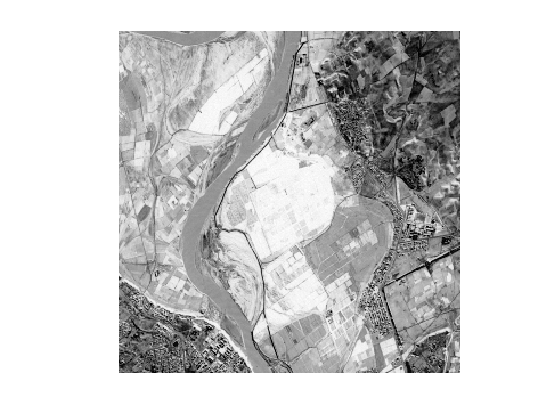Set options for the propagation: infinite number of iterations, and stop when the front hits the end point.

options.nb_iter_max = Inf;
options.end_points = x1;


Perform the propagation, so that $$D(a,b)$$ is the geodesic distance between the pixel $$x_1=(a,b)$$ and the starting point $$x_0$$. Note that the function perform_fast_marching takes as input the inverse of the metric $$1/W(x)$$.

[D,S] = perform_fast_marching(1./W, x0, options);


Display the propagated distance map $$D$$. We display in color the distance map in areas where the front has propagated, and leave in black and white the area where the front did not propagate.

clf;
hold on;
imageplot( convert_distance_color(D,f) );
h = plot(x0(2),x0(1), '.r'); set(h, 'MarkerSize', 25);
h = plot(x1(2),x1(1), '.b'); set(h, 'MarkerSize', 25);Exercice 1: (check the solution) Using options.nb_iter_max, display the progressive propagation. This corresponds to displaying the front $$\enscond{x}{D(x) \leq t}$$ for various arrival times $$t$$.

exo1;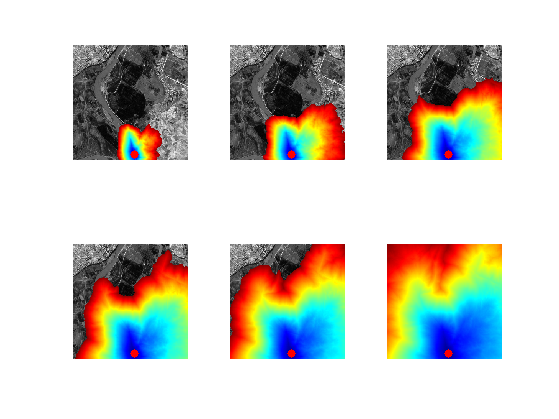## Geodesic Curve Extraction

Once the geodesic distance map $$D(x)$$ to a starting point $$x_0$$ is computed, the geodesic curve between any point $$x_1$$ and $$x_0$$ extracted through gradient descent $\ga'(t) = - \eta_t \nabla D(\ga(t)),$ where $$\eta_t>0$$ controls the parameterization speed of the resulting curve. To obtain unit speed parameterization, one can use $$\eta_t = \norm{\nabla D(\ga(t))}^{-1}$$.

Recompute the geodesic distance map $$D$$ on the whole grid.

options.nb_iter_max = Inf;
options.end_points = [];
[D,S] = perform_fast_marching(1./W, x0, options);


Display $$D$$.

clf;
imageplot(D);
colormap jet(256);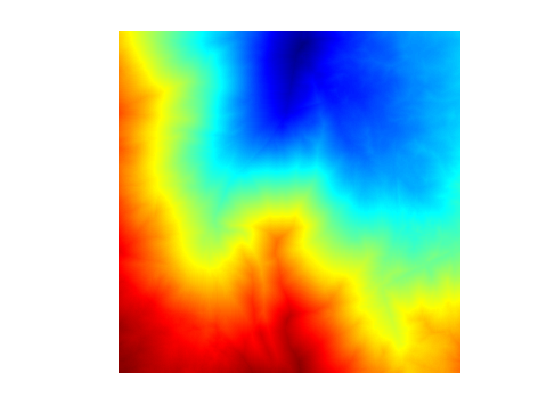Compute the gradient $$G_0(x) = \nabla D(x) \in \RR^2$$ of the distance map. Use centered differences.

options.order = 2;
G0 = grad(D, options);


Normalize the gradient to obtained $$G(x) = G_0(x)/\norm{G_0(x)}$$, in order to have unit speed geodesic curve (parameterized by arc length).

G = G0 ./ repmat( sqrt( sum(G0.^2, 3) ), [1 1 2]);


Display $$G$$.

clf;
imageplot(G);
colormap jet(256);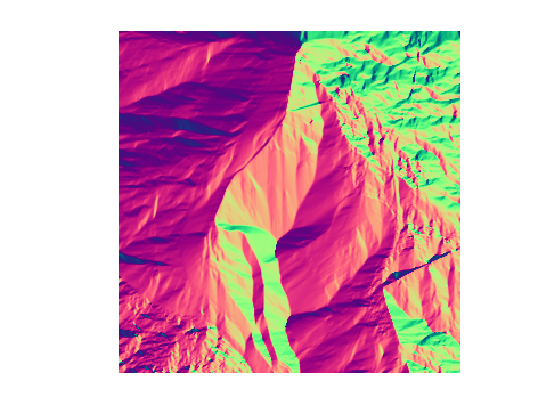The geodesic is then numerically computed using a discretized gradient descent, which defines a discret curve $$(\ga_k)_k$$ using $\ga_{k+1} = \ga_k - \tau G(\ga_k)$ where $$\ga_k \in \RR^2$$ is an approximation of $$\ga(t)$$ at time $$t=k\tau$$, and the step size $$\tau>0$$ should be small enough.

Step size $$\tau$$ for the gradient descent.

tau = .8;


Initialize the path with the ending point.

gamma = x1;


Define a shortcut to interpolate $$G$$ at a 2-D points. Warning: the interp2 switches the role of the axis ...

Geval = @(G,x)[interp2(1:n,1:n,G(:,:,1),x(2),x(1)); ...
interp2(1:n,1:n,G(:,:,2),x(2),x(1)) ];


Compute the gradient at the last point in the path, using interpolation.

g = Geval(G, gamma(:,end));


Perform the descent and add the new point to the path.

gamma(:,end+1) = gamma(:,end) - tau*g;


Exercice 2: (check the solution) Perform the full geodesic path extraction by iterating the gradient descent. You must be very careful when the path become close to $$x_0$$, because the distance function is not differentiable at this point. You must stop the iteration when the path is close to $$x_0$$.

exo2;


Display the curve on the image background.

clf; hold on;
imageplot(f);
h = plot(gamma(2,:),gamma(1,:), '.b'); set(h, 'LineWidth', 2);
h = plot(x0(2),x0(1), '.r'); set(h, 'MarkerSize', 25);
h = plot(x1(2),x1(1), '.b'); set(h, 'MarkerSize', 25);
axis ij;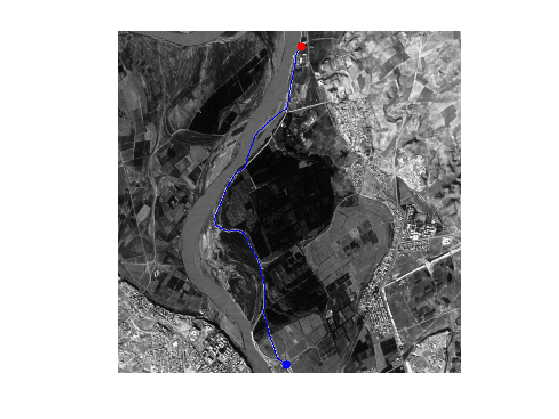Display the curve on the distance background.

clf; hold on;
imageplot(D); colormap jet(256);
h = plot(gamma(2,:),gamma(1,:), '.b'); set(h, 'LineWidth', 2);
h = plot(x0(2),x0(1), '.r'); set(h, 'MarkerSize', 25);
h = plot(x1(2),x1(1), '.b'); set(h, 'MarkerSize', 25);
axis ij;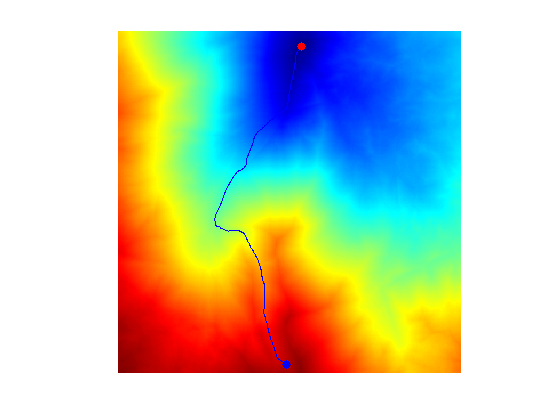Exercice 3: (check the solution) Study the influence of the $$\epsilon$$ parameter.

exo3;Exercice 4: (check the solution) Perform the shortest path extraction for various images such as 'cavern' or 'mountain'.

exo4;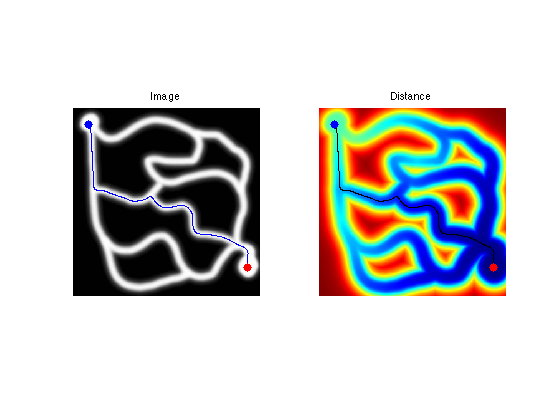## Edge-based Geodesic Metric

It is possible to extract the boundary of an object using shortest paths that follows region of high gradient.

First we load an image $$f$$.

n = 256;
name = 'cortex';
f = rescale( sum(load_image(name,n),3) );


Display it.

clf;
imageplot(f);An edge-attracting potential $$W(x)$$ should be small in regions of high gradient. A popular choice is $W(x) = \frac{1}{\epsilon + G_\si \star G(x)} \qwhereq G(x) = \norm{\nabla f(x)},$ and where $$G_\si$$ is a Gaussian kernel of variance $$\si^2$$.

Compute the gradient norm $$G(x)$$.

G = grad(f,options);
G = sqrt( sum(G.^2,3) );


Smooth it by $$G_\si$$.

sigma = 3;
Gh = perform_blurring(G,sigma);


Display the smoothed gradient $$G \star G_\si$$.

clf;
imageplot(Gh);Compute the metric.

epsilon = 0.01;
W = 1./( epsilon + Gh );


Display it.

clf;
imageplot(W);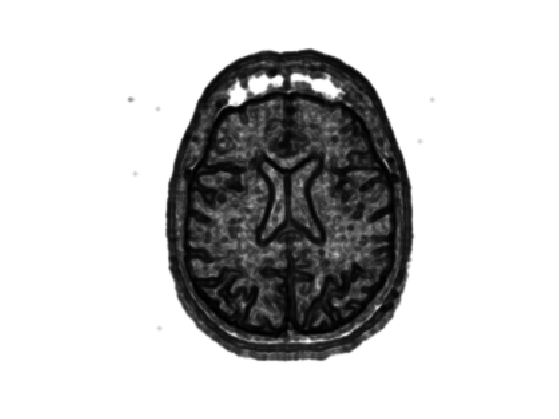Set two starting point $$\Ss = \{x_0^1,x_0^2\}$$ (you can use other points).

x0 = [ [136;53] [123;205]];


Compute the Fast Marching from these two base points.

options.nb_iter_max = Inf;
options.end_points = [];
[D,S,Q] = perform_fast_marching(1./W, x0, options);


Display the geodesic distance (with color normalization).

clf; hold on;
imageplot( perform_hist_eq(D,'linear') );
h = plot(x0(2,:),x0(1,:), '.r'); set(h, 'MarkerSize', 25);
colormap jet(256);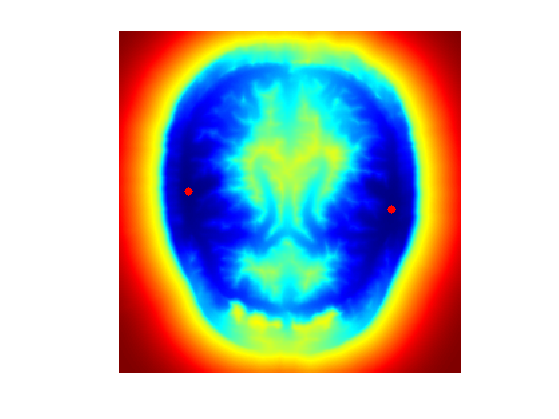The Voronoi segmentation associated to $$\Ss$$ is $\Cc_i = \enscond{x}{ \forall j \neq i, \; d(x_0^i,x) \leq d(x_0^j,x) }.$

This Voronoi segmentation is computed during the Fast Marching propagation and is encoded in the partition function $$Q(x)$$ using $$\Cc_i = \enscond{x}{Q(x)=i}$$.

Display the distance and the Voronoi segmentation.

clf; hold on;
A = zeros(n,n,3); A(:,:,1) = rescale(Q); A(:,:,3) = f;
imageplot(A);
h = plot(x0(2,:),x0(1,:), '.g'); set(h, 'MarkerSize', 25);Exercice 5: (check the solution) Extract the set of points that are along the boundary of the Voronoi region. This corresponds for instance to the points of the region $$\enscond{x}{Q(x)=1}$$ that have one neighbor inside the region $$\enscond{x}{Q(x)=2}$$. Compute the geodesic distance $$D(x)$$ at these points, and choose two points $$a$$ and $$b$$ on this boundary that have small values of $$D$$.

exo5;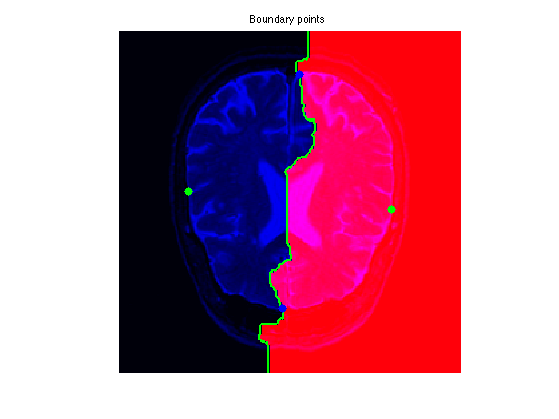Exercice 6: (check the solution) Extract the geodesics joining $$a$$ and $$b$$ to the two starting points (this makes 4 geodesic curves). Use them to perform segmentation.

exo6;## Vessel Segmentation and Centerline Extraction

One can extract a network of geodesic curve starting from a central point to detect vessels in medical images.

Load an image. This image is extracted from the DRIVE database of retinal vessels.

n = 256;
name = 'vessels';
f = rescale(load_image(name, n));


Display it.

clf;
imageplot(f);We clean the image by substracting the smoothly varying background $f_1 = f - G_\si \star f,$ where $$G_\si$$ is a Gaussian kernel of variance $$\si^2$$. Computing $$f_1$$ corresponds to a high pass filtering.

sigma = 20;
f1 = perform_blurring(f,sigma) - f;


Display this normalized image.

clf;
imageplot(f1);We compute a metric tthat is small for large values of $$f_1$$: $W(x) = \epsilon + \abs{f_1(x)-c} \qwhereq c = \umax{x} f_1(x).$

c = max(f1(:));
epsilon = 1e-2;
W = epsilon + abs(f1-c);


Display the metric.

clf,
imageplot(W);Select a central point $$x_0$$ for the network.

x0 = [142;226];


Exercice 7: (check the solution) Perform partial propagations from $$x_0$$.

exo7;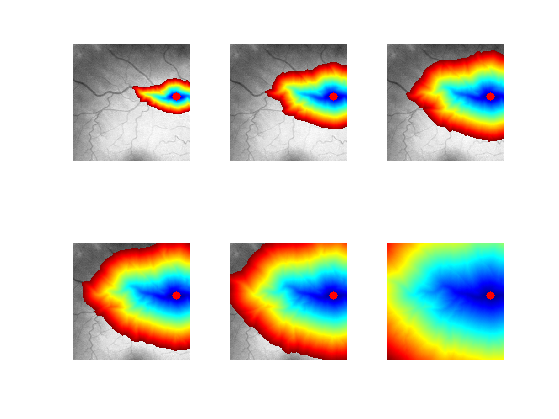Exercice 8: (check the solution) Extract geodesics joining several points $$x_1$$ to the central point $$x_0$$.

exo8;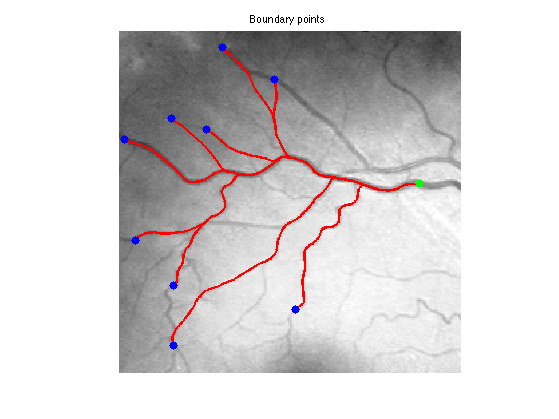## Dual Propagation

In order to speed up geodesic extraction, one can perform the propagation from both the start point $$x_0^1$$ and end point $$x_0^2$$.

Boundary points.

x0 = [[143;249] [174;9]];


Exercice 9: (check the solution) Perform the dual propagation, and stop it when the front meet. Extract the two half geodesic curves.

exo9;